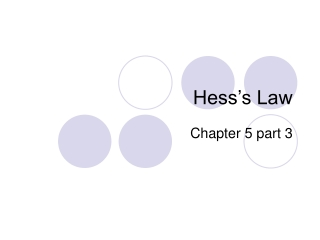DownloadDownload PresentationHess’s Law

# Hess’s Law

Télécharger la présentation## Hess’s Law

- - - - - - - - - - - - - - - - - - - - - - - - - - - E N D - - - - - - - - - - - - - - - - - - - - - - - - - - -
##### Presentation Transcript

1. Hess’s Law Chapter 5 part 3

2. Enthalpy is a state function, therefore: • In going from a particular set of reactants to a particular set of products, the change in enthalpy is the same whether the reaction takes place in one step or a series of steps. Hess’s Law

3. Since ΔH is a state function… • It doesn’t matter the route. In any reaction the change in enthalpy is the same whether the reaction takes place in one step or in a series of steps. • Therefore, one may sum up the enthalpies of a series of reaction just as one adds up a series of reactions. • But…, if the reaction is reversed, so is the sign of the enthalpy. • If the reaction is multiplied, so is the enthalpy.

4. Some ΔH’s cannot be measured in a Calorimeter: • Example: • C graphite C diamond • Yes this reaction is real!! • This cannot be measured directly. • Why???

5. But using Hess’s Law, it can be calculated: • Take the heats of combustion for each: • C graphite+ O2(g) CO2 (g) ΔH = - 394 kj • C diamond + O2(g) CO2 (g) ΔH = -396 kj • If you reverse the second: • CO2(g)  C diamond+ O2(g)ΔH = +396 kj

6. Hess’s Law of Summation • CO2(g)  C diamond+ O2(g)ΔH = +396 kj • C graphite + O2(g) CO2 (g) ΔH = -394 kj • Equals • C graphite C diamond ΔH = +2 kj • Is this reaction endothermic • or exothermic?

7. Worksheet!!!